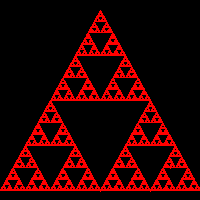# imagesetpixel

(PHP 4, PHP 5)

imagesetpixel βÄî Set a single pixel

### Description

bool imagesetpixel ( resource \$image , int \$x , int \$y , int \$color )

imagesetpixel() draws a pixel at the specified coordinate.

### Parameters

image

An image resource, returned by one of the image creation functions, such as imagecreatetruecolor().

x

x-coordinate

y

y-coordinate

color

A color identifier created with imagecolorallocate()

### Return Values

Returns TRUE on success or FALSE on failure.

### Examples

Example#1 imagesetpixel() example

A random drawing that ends with a regular picture.

``` <?php\$x = 200;\$y = 200;\$gd = imagecreatetruecolor(\$x, \$y); \$corners = array('x' => 100, 'y' =>  10);\$corners = array('x' =>   0, 'y' => 190);\$corners = array('x' => 200, 'y' => 190);\$red = imagecolorallocate(\$gd, 255, 0, 0); for (\$i = 0; \$i < 100000; \$i++) {  imagesetpixel(\$gd, round(\$x),round(\$y), \$red);  \$a = rand(0, 2);  \$x = (\$x + \$corners[\$a]['x']) / 2;  \$y = (\$y + \$corners[\$a]['y']) / 2;} header('Content-Type: image/png');imagepng(\$gd);?> ```

The above example will output something similar to: